# User:MiloKing/SalesTaxEdit

## Effect of sales tax on a single good with a monopolist-controlled market

We consider comparative statics between two situations:

• A world where there are no sales taxes
• A world where sales taxes are introduced on a single good (or class of goods) for which we are drawing the supply and demand curves.

Note that the same analysis also works for comparative statics where we change the sales tax on only one class of goods.

Unlike in the previous section, here we assume that the market for the single good is supplied solely by one firm which is seeking to maximize its profits. Here, the demand curve the firm faces for its product is the same as the demand curve for the industry as a whole, so the price they receive for their goods declines as the quantity sold increases (assuming the law of demand holds). This means that the marginal revenue from selling a unit is lower than the price of that unit. Since maximizing profits involves setting marginal cost equal to marginal revenue, the monopolist will produce goods at a level where price exceeds the marginal cost of producing a good.

The marginal revenue from the sale of a good equals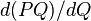$d(PQ)/dQ$, as$PQ$ is revenue and we are interested in the rate of change of revenue as quantity changes. Using the Product Rule of calculus, we find that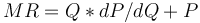$MR = Q*dP/dQ + P$. As explained previously, we expect that dP/dQ is negative in accordance with the law of demand. As such, marginal revenue is less than the price paid, and it may even be zero or negative.

Furthermore, we avoid referring to a "supply curve" for a monopolist and instead refer to its "marginal cost curve". The latter refers to the relationship between the quantity of the good the monopolist produces and the marginal cost of producing each unit. The reason we do not call this the supply curve is that at the level of quantity traded, price exceeds marginal cost, and so the demand curve does not intersect the marginal cost curve at this point. Calling the marginal cost curve the "supply curve" would imply that it should, as supply and demand curves are normally expected to intersect when the market is in equilibrium.

### Analytical tools

There are three kinds of diagrams that we draw to study the situation:

1. Consider the world without sales tax. We can draw the usual marginal cost, marginal revenue, and demand curves and do the usual analysis to find the market price and equilibrium quantity traded.
2. Consider the world with sales tax. In this world, consider marginal cost, marginal revenue, and demand curves drawn with respect to pre-tax prices.
3. Consider the world with sales tax. In this world, consider supply, marginal revenue, and demand curves with respect to post-tax prices.

### Analysis with pre-tax prices

We want to perform comparative statics between the world without sales tax and the world with sales tax. We consider the marginal cost, demand, and marginal revenue curves for the latter in terms of the pre-tax prices.

Marginal cost curve: The marginal cost curve using pre-tax prices is expected to remain the same as the marginal cost curve in a world without taxes, because the price that the seller sees is the pre-tax price.

Demand curve: The demand curve changes. In general, it moves downward. Assuming the law of demand, that is the same as moving inward, i.e., a contraction of the demand curve. Arithmetically, the new demand curve is related to the old demand curve as follows:

Case What happens to the demand curve algebraically Pictorial depiction
price-propotional sales tax For a price-proportional sales tax with a factor of$x$, the new demand function at a price of$p$ equals the old demand function at a price of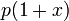$p(1 + x)$. The reason is that, as far as buyers are concerned, the effective price is$p(1 + x)$. The upshot is that the demand curve shrinks downward by a factor of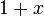$1 + x$. An example picture is below, comparing the no-tax demand curve (solid blue) with the pre-tax demand curve for a 50% sales tax (dashed purple). The original curve shrinks downward to 2/3 of its original level.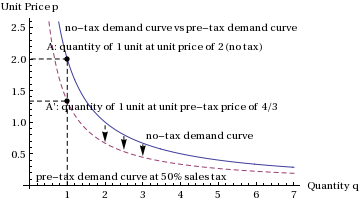quantity-proportional sales tax For a quantity-proportional sales tax with a tax of$b$ per unit quantity, the new demand function at a price of$p$ is the old demand function at a price of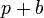$p + b$. The reason is that, as far as buyers are concerned, the effective price is$p + b$. The upshot is that the demand curve shifts downward by a vertical distance of$b$. An example picture is below, comparing the no-tax demand curve (solid blue) with the pre-tax demand curve for a 0.4 price units/unit quanity sales tax (dashed purple). The original curve shrinks downward by 0.4 price units from its original level.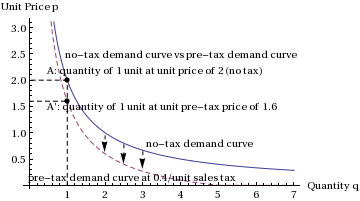Marginal revenue curve: The marginal revenue curve changes. In general, it moves downward in the case of a quantity-proportional sales tax, and closer to zero in the case of a price-proportional sales tax. (This means that in the case of a price-proportional sales tax, if marginal revenue is negative at a given quantity sold, marginal revenue will actually increase at that point, as it will be less negative.) Assuming the law of demand, the marginal revenue curve slopes downwards, so that is the same as moving inward, i.e., a contraction of the demand curve. Arithmetically, the new marginal revenue curve is related to the old marginal revenue curve as follows:

Case What happens to the marginal revenue curve algebraically Pictorial depiction
price-propotional sales tax For a price-proportional sales tax with a factor of$x$, the new marginal revenue function equals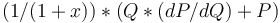$(1/(1+x))*(Q*(dP/dQ) + P)$. The reason is that the demand curve is multiplied by 1/(1+x), so instead of marginal revenue equaling$d(PQ)/dQ$, it now equals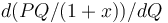$d(PQ/(1+x))/dQ$. An example picture is below, comparing the no-tax marginal revenue curve (solid blue) with the pre-tax marginal revenue curve for a 50% sales tax (dashed purple). The original curve shrinks downward to 2/3 of its original level.
INSERT IMAGE
quantity-proportional sales tax For a quantity-proportional sales tax with a tax of$b$ per unit quantity, the new marginal revenue function equals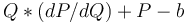$Q*(dP/dQ) + P - b$. The reason is that the demand curve shifts downward by b, so instead of marginal revenue equaling$d(PQ)/dQ$, it now equals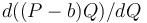$d((P-b)Q)/dQ$. An example picture is below, comparing the no-tax marginal revenue curve (solid blue) with the pre-tax marginal revenue curve for a 0.4 price units/unit quanity sales tax (dashed purple). The original curve shrinks downward by 0.4 price units from its original level. INSERT IMAGE

The upshot is that the marginal cost curve remains the same and the demand and marginal revenue curves move inward. We can therefore determine that the equilibrium quantity traded falls. However, we cannot necessarily determine whether the pre-tax price will rise or fall. In most cases, we would expect the pre-tax price to fall, as demand falls. However, it is possible for the pre-tax price to rise if the monopolist switches from one potential equilibrium to another.

To illustrate how this latter possibility could happen, imagine that one buyer of the good is willing to pay \$3000 for a single unit while all subsequent buyers are willing to pay \$2 per unit (up to the 10,000th unit). Imagine also that the marginal cost of producing the first unit is \$500, while the marginal cost of producing all units thereafter is \$1. The marginal revenue for the first unit is \$3000, for the second unit is -\$2996 (as the seller would get \$2 for each of the two units sold but would effectively lose the ability to charge \$3000 for the first unit), and for all units thereafter is \$2. With no tax in place, the monopolist would choose to sell 10,000 units for \$2 each, making a profit of \$9501 (\$20,000 - \$9,999 - \$500) rather than only selling one unit for \$3000 (which would only make a profit of \$2500). Now suppose a 300% sales tax is imposed. This effectively reduces all marginal revenue figures by 3/4ths. Selling units to customers only willing to pay \$2 per unit would no longer be possible, as the pre-tax price could be \$0.50 at maximum, and the marginal cost of producing units is \$1. However, the buyer willing to pay \$3000 could pay a pre-tax price of \$750 and the monopolist could make a profit of \$250 selling to that buyer alone. So the result of the sales tax in this (unusual) case would be to raise the pre-tax price of the unit from \$2 to \$750.

### Analysis with post-tax prices

To analyze the imposition of a sales tax on a monopolistic market, it is easiest to take the analysis for pre-tax prices and simply apply the sales tax on top of those prices. The net effect of the tax on the equilibrium quantity traded is negative. The net effect of the tax on the post-tax price is zero or positive. (Even in the case where the pre-tax price falls, it cannot fall by more than the level of the tax imposed. At maximum, the pre-tax price will fall by the full amount of the tax; this happens in the case where the marginal cost curve is completely inelastic.)

### Combined analysis and conclusions

Combining both these analyses, we obtain the three conclusions:

• The pre-tax market price may be lower or higher than the market price in a world without the tax (this can be seen via the comparative statics between the no-tax and the pre-tax curves)
• The post-tax market price is higher than the market price in a world without the tax (this can be seen via the comparative statics between the no-tax and the post-tax curves)
• The equilibrium quantity traded is less than the equilibrium quantity traded in a world without the tax (this can be seen using either of the two comparative statics methods employed above)

### Extreme cases of elastic and inelastic supply and demand

We consider some extreme cases. The first row describes the standard case, and subsequent rows describe extreme cases:

Assumption for price-elasticity of demand Assumption for price-elasticity of marginal cost Conclusion about pre-tax market price (relative to market price in a world without the tax) Conclusion about post-tax market price (relative to market price in a world without the tax) Conclusion about equilibrium quantity traded (relative to a world without the tax)
negative (satisfies the law of demand) positive (satisfies the law of supply) indeterminate rises falls
infinite, i.e., a horizontal demand curve (e.g., when the good has a perfect substitute) positive (satisfies the law of supply) indeterminate stays the same falls
zero, i.e., a vertical demand curve. We also say that the demand is perfectly price-inelastic positive (satisfies the law of supply) indeterminate rises stays the same
negative (satisfies the law of demand) infinite, i.e., a horizontal supply curve, e.g., a constant cost industry. Alternatively, this also applies if the jurisdiction where sales tax is imposed is a small subjurisdiction of the economy and the pre-tax prices of the goods are determined by the world economy. indeterminate rises falls
negative (satisfies the law of demand) zero, i.e., a vertical supply curve. We say that the supply is perfectly price-inelastic indeterminate stays the same stays the same

### How price-elasticity affects the nature of the effect of sales tax

The extent to which the sales tax affects the pre-tax price, post-tax price, and equilibrium quantity traded depends upon the price-elasticity of demand and price-elasticity of supply. The following are two general principles:

• Between demand and supply, the relatively more price-inelastic side absorbs more of the price burden of the sales tax. In other words, if demand is more inelastic, then the effect of the sales tax is largely seen in terms of an increase in the post-tax price. If supply is more inelastic, then the effect of the sales tax is largely seen in terms of a decrease in the pre-tax price. This is in keeping with the general principle attributed to Ricardo that rents are captured by the most inelastic side. Here, the rents are reversed in sign, but the principle stays the same.
• In general, the extent to which the equlibrium quantity traded is affected is negatively related to the price-elasticities of both demand and supply. In other words, if we reduce the price-elasticity of either demand or supply, the sensitivity of the equilibrium quantity traded to the sales tax reduces. In particular, if either demand or supply is perfectly price-inelastic, the equilibrium quantity traded is independent of the sales tax.